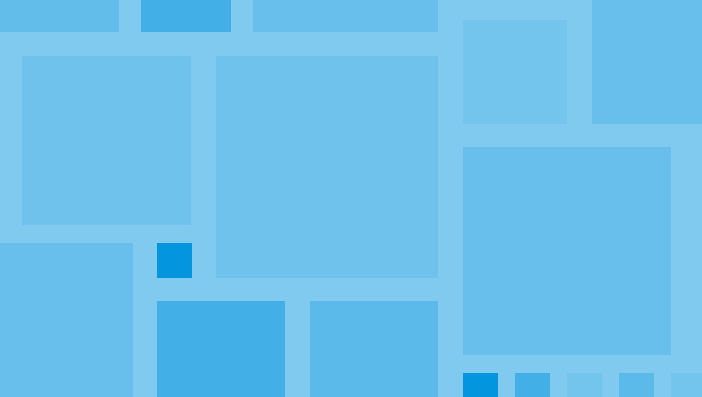# An introduction to quantum computingWho
Yvan Le Borgne
Département Combinatoire et Algorithmique, LABRI, Université Bordeaux & CNRS

Where
CFM Auditorium

When
6, 7 and 8 June 2023, 10h30 – 12h00

Objectives:

This lecture introduces the elementary notions of quantum computation at the scales usually known by a computer scientist that is from the notions of quantum bits, gates and circuits up to the algorithms solving problems.
We briefly discuss the possible physical devices able to support the basic elements.
Then we check how the classical computation may be seen as a subset of quantum computation.
We also study quantum algorithms significantly more efficient (on an ideal quantum computer) than classical algorithms for some problems due to Deutsh-Josza, Simon and Grover.
We present the BB84 protocol for quantum key distribution that relies on no-cloning theorem.
We briefly illustrate the possibility of programming and simulating quantum circuits.

Outline:

Quantum computation revisits with interference and entanglement the chain supporting computation on classical computers that goes from physical devices to algorithms solving problems. The aim of this lecture is to give a quick overview of this field. A tentative outline is :

• What is a quantum bit? A quantum gate? A quantum circuit?
• An interferometer’s experiment dating from XIXth century as first physical quantum circuit not explainable by probabilities.
• What are the possible physical devices supporting quantum computation and their current qualities? (sketched )
• Despite the restrictions on qubits, especially reversibility of computation before measurement, does quantum circuits extend classical circuits? (yes, and we will see a proof may be not so efficient but accessible).
• What are the good old problems (some of them from the 90’s) significantly better solved on an hypotetic perfect quantum computer than on an (also perfect) classical computer?
• How to program today targeting a quantum computer?
• The not detailed Shor’s algorithm factoring integers is a threat for cryptography based on RSA, but is the no-cloning theorem a decisive advantage for quantum key distribution? (BB84 protocol)
• How to improve qualities of quantum circuits via software? (Quantum error correction, Threshold’s theorem)

Since the pace of the lecture will by adapted to the reaction of the audience, if time permits:

• How to get lower bounds on complexity for quantum circuits solving a problem? (especially using the polynomial method)
• Quantum phase estimation
• What is a universal set of gates for quantum circuits? (compare to classical circuits)
• What is new in compilation of quantum circuits?

Skills acquired during lecture:

What are quantum bits, gates, circuits and their measurement.
Superposition in qubit’s states with possibility of interference.
Various physical devices for qubits with an intuition of their advantages and drawbacks.
How any boolean function is computable via quantum circuit.
Deutsch-Jozsa’s algorithm, Simon’s algorithms, Grover’s algorithm.
Describing quantum circuit in python and simulating their execution.
(Idealized) BB84 protocol for quantum key distribution based on no-cloning theorem.